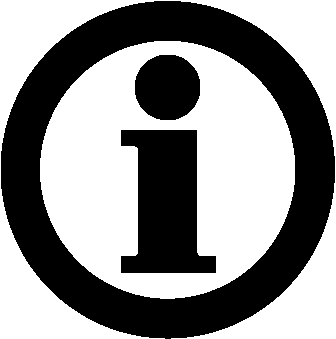## Conversion Tables

Metric Units of Length

1 millimeter (mm) = 1/1,000 meter
1 centimeter (cm) = 1/100 meter
1 decimeter (dm) = 1/10 meter
1 meter (m; basic unit of length)
1 dekameter (dkm) = 10 meters
1 kilometer (km) = 1000 meters

Conversion Factors for Units of Length

1 inch = 2.54 centimeters
1 foot = 0.305 meter
1 yard = 0.914 meter
1 mile = 1.609 kilometers
1 nautical mile = 1.852 kilometers
1 centimeter = 0.39 inch
1 meter = 39.37 inches = 3.28 feet = 1.094 yards
1 kilometer = 0.62 mil

Conversion Factors for Units of Volume & Capacity

1 cubic inch = 16.4 cubic centimeters
1 cubic foot = 0.0283 cubic meter
1 cubic yard = 0.765 cubic meter
1 fluid ounce = 29.6 milliliters
1 U.S. pint = 0.473 liter
1 U.S. quart = 0.946 liter
1 U.S. gallon = 0.84 imperial gallon = 3.8 liters
1 imperial gallon = 1.2 U.S. gallons = 4.5 liters
1 dry pint = 0.55 liters
1 dry quart = 1.1 liters
1 U.S. bushel = 0.97 imperial bushel = 35.24 liters
1 imperial bushel = 1.03 U.S. bushels = 36.37 liters
1 cubic centimeter = 0.06 cubic inch
1 cubic centimeter = 0.06 cubic inch
1 cubic meter = 1.3 cubic yards
1 milliliter = 0.034 fluid ounce
1 liter = 1.06 U.S.quarts = 0.9 dry quart
1 dekaliter = 0.28 U.S. bushel